Tutorials
machine learning
+1

# Decision Trees in R

Learn all about decision trees, a form of supervised learning used in a variety of ways to solve regression and classification problems.Let's imagine you are playing a game of Twenty Questions. Your opponent has secretly chosen a subject, and you must figure out what he/she chose. At each turn, you may ask a yes-or-no question, and your opponent must answer truthfully. How do you find out the secret in the fewest number of questions?

It should be obvious some questions are better than others. For example, asking "Can it fly?" as your first question is likely to be unfruitful, whereas asking "Is it alive?" is a bit more useful. Intuitively, you want each question to significantly narrow down the space of possibly secrets, eventually leading to your answer.

That is the basic idea behind decision trees. At each point, you consider a set of questions that can partition your data set. You choose the question that provides the best split and again find the best questions for the partitions. You stop once all the points you are considering are of the same class. Then the task of classication is easy. You can simply grab a point, and chuck it down the tree. The questions will guide it to its appropriate class.

Since this tutorial is in R, I highly recommend you take a look at our Introduction to R or Intermediate R course, depending on your level of advancement.

## Introduction

Decision tree is a type of supervised learning algorithm that can be used in both regression and classification problems. It works for both categorical and continuous input and output variables.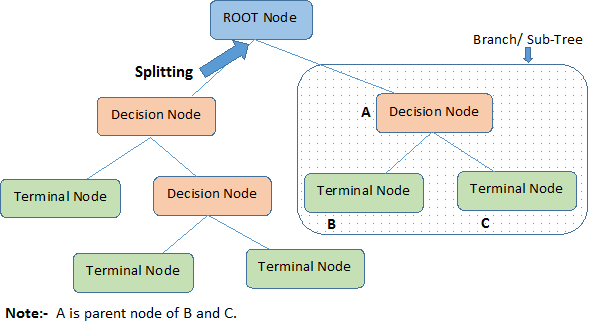Let's identify important terminologies on Decision Tree, looking at the image above:

• Root Node represents the entire population or sample. It further gets divided into two or more homogeneous sets.

• Splitting is a process of dividing a node into two or more sub-nodes.

• When a sub-node splits into further sub-nodes, it is called a Decision Node.

• Nodes that do not split is called a Terminal Node or a Leaf.

• When you remove sub-nodes of a decision node, this process is called Pruning. The opposite of pruning is Splitting.

• A sub-section of an entire tree is called Branch.

• A node, which is divided into sub-nodes is called a parent node of the sub-nodes; whereas the sub-nodes are called the child of the parent node.

## Types of Decision Trees

### Regression Trees

Let's take a look at the image below, which helps visualize the nature of partitioning carried out by a Regression Tree. This shows an unpruned tree and a regression tree fit to a random dataset. Both the visualizations show a series of splitting rules, starting at the top of the tree. Notice that every split of the domain is aligned with one of the feature axes. The concept of axis parallel splitting generalises straightforwardly to dimensions greater than two. For a feature space of size $p$, a subset of $\mathbb{R}^p$, the space is divided into $M$ regions, $R_{m}$, each of which is a $p$-dimensional "hyperblock".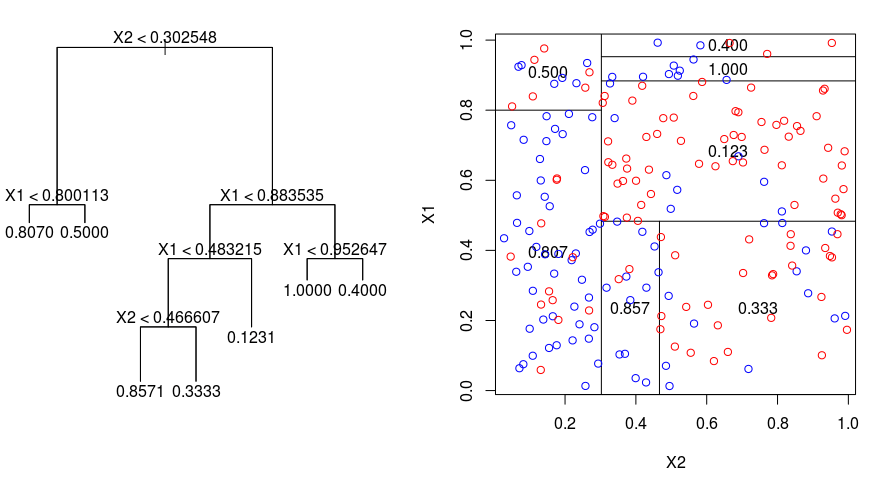In order to build a regression tree, you first use recursive binary splititng to grow a large tree on the training data, stopping only when each terminal node has fewer than some minimum number of observations. Recursive Binary Splitting is a greedy and top-down algorithm used to minimize the Residual Sum of Squares (RSS), an error measure also used in linear regression settings. The RSS, in the case of a partitioned feature space with M partitions is given by: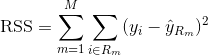Beginning at the top of the tree, you split it into 2 branches, creating a partition of 2 spaces. You then carry out this particular split at the top of the tree multiple times and choose the split of the features that minimizes the (current) RSS.

Next, you apply cost complexity pruning to the large tree in order to obtain a sequence of best subtrees, as a function of $\alpha$. The basic idea here is to introduce an additional tuning parameter, denoted by $\alpha$ that balances the depth of the tree and its goodness of fit to the training data.

You can use K-fold cross-validation to choose $\alpha$. This technique simply involves dividing the training observations into K folds to estimate the test error rate of the subtrees. Your goal is to select the one that leads to the lowest error rate.

### Classification Trees

A classifiction tree is very similar to a regression tree, except that it is used to predict a qualitative response rather than a quantitative one.

Recall that for a regression tree, the predicted response for an observation is given by the mean response of the training observations that belong to the same terminal node. In contrast, for a classification tree, you predict that each observation belongs to the most commonly occurring class of training observations in the region to which it belongs.

In interpreting the results of a classification tree, you are often interested not only in the class prediction corresponding to a particular terminal node region, but also in the class proportions among the training observations that fall into that region.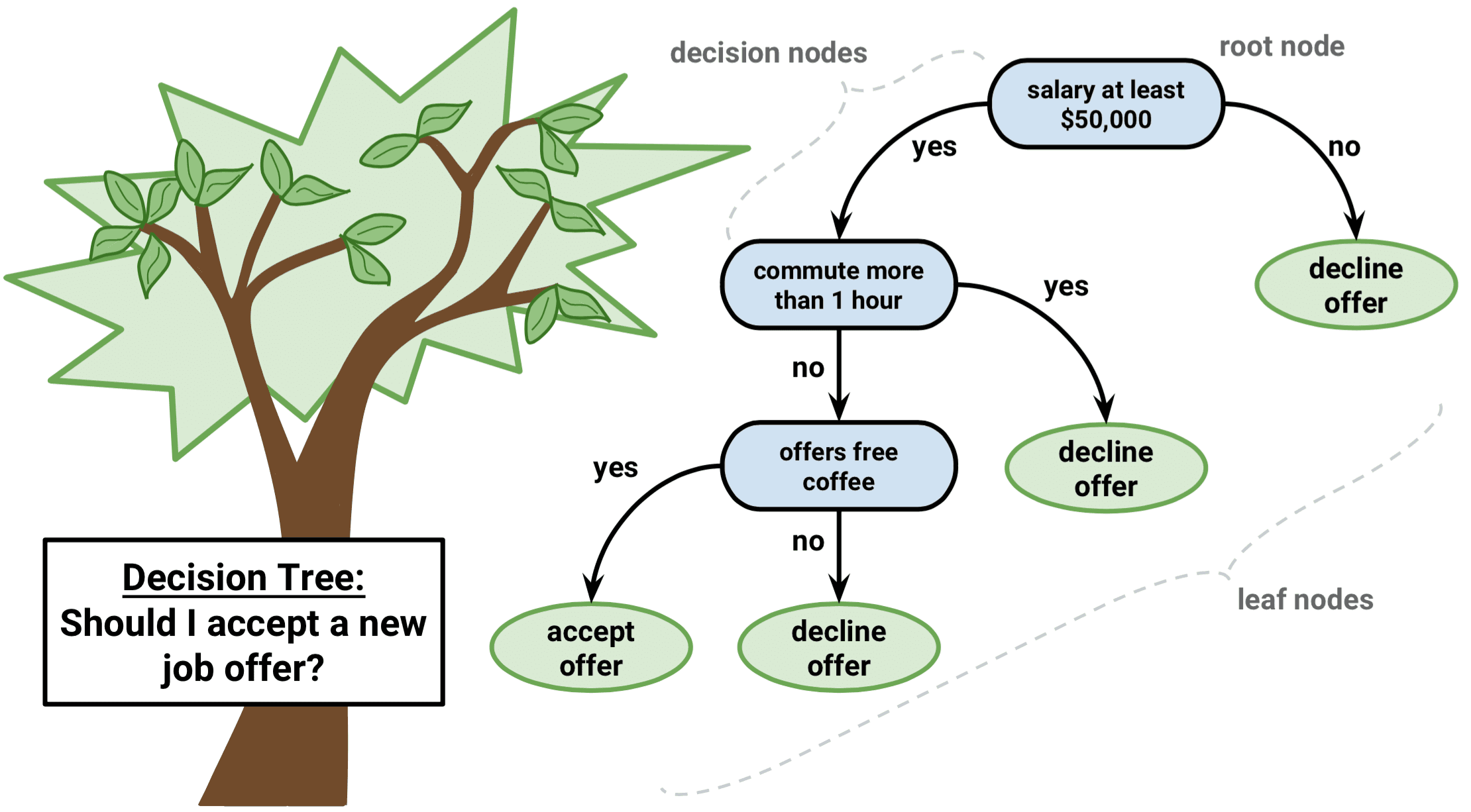The task of growing a classification tree is quite similar to the task of growing a regression tree. Just as in the regression setting, you use recursive binary splitting to grow a classification tree. However, in the classification setting, Residual Sum of Squares cannot be used as a criterion for making the binary splits. Instead, you can use either of these 3 methods below:

• Classification Error Rate: Rather than seeing how far a numerical response is away from the mean value, as in the regression setting, you can instead define the "hit rate" as the fraction of training observations in a particular region that don't belong to the most widely occuring class. The error is given by this equation:

E = 1 - argmaxc($\hat{\pi}_{mc}$)

in which $\hat{\pi}_{mc}$ represents the fraction of training data in region Rm that belong to class c.

• Gini Index: The Gini Index is an alternative error metric that is designed to show how "pure" a region is. "Purity" in this case means how much of the training data in a particular region belongs to a single class. If a region Rm contains data that is mostly from a single class c then the Gini Index value will be small: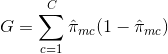• Cross-Entropy: A third alternative, which is similar to the Gini Index, is known as the Cross-Entropy or Deviance:The cross-entropy will take on a value near zero if the $\hat{\pi}_{mc}$’s are all near 0 or near 1. Therefore, like the Gini index, the cross-entropy will take on a small value if the mth node is pure. In fact, it turns out that the Gini index and the cross-entropy are quite similar numerically.

When building a classification tree, either the Gini index or the cross-entropy are typically used to evaluate the quality of a particular split, since they are more sensitive to node purity than is the classification error rate. Any of these 3 approaches might be used when pruning the tree, but the classification error rate is preferable if prediction accuracy of the final pruned tree is the goal.

The major advantage of using decision trees is that they are intuitively very easy to explain. They closely mirror human decision-making compared to other regression and classification approaches. They can be displayed graphically, and they can easily handle qualitative predictors without the need to create dummy variables.

However, decision trees generally do not have the same level of predictive accuracy as other approaches, since they aren't quite robust. A small change in the data can cause a large change in the final estimated tree.

By aggregating many decision trees, using methods like bagging, random forests, and boosting, the predictive performance of decision trees can be substantially improved.

## Tree-Based Methods

### Bagging

The decision trees discussed above suffer from high variance, meaning if you split the training data into 2 parts at random, and fit a decision tree to both halves, the results that you get could be quite different. In contrast, a procedure with low variance will yield similar results if applied repeatedly to distinct dataset.

Bagging, or bootstrap aggregation, is a technique used to reduce the variance of your predictions by combining the result of multiple classifiers modeled on different sub-samples of the same dataset. Here is the equation for bagging: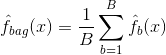in which you generate $B$ different bootstrapped training datasets. You then train your method on the $bth$ bootstrapped training set in order to get $\hat{f}_{b}(x)$, and finally average the predictions.

The visual below shows the 3 different steps in bagging: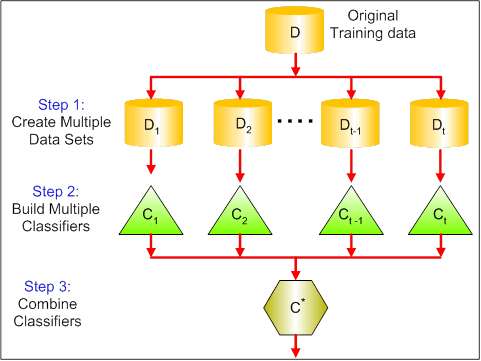• Step 1: Here you replace the original data with new data. The new data usually have a fraction of the original data's columns and rows, which then can be used as hyper-parameters in the bagging model.

• Step 2: You build classifiers on each dataset. Generally, you can use the same classifier for making models and predictions.

• Step 3: Lastly, you use an average value to combine the predictions of all the classifiers, depending on the problem. Generally, these combined values are more robust than a single model.

While bagging can improve predictions for many regression and classification methods, it is particularly useful for decision trees. To apply bagging to regression/classification trees, you simply construct $B$ regression/classification trees using $B$ bootstrapped training sets, and average the resulting predictions. These trees are grown deep, and are not pruned. Hence each individual tree has high variance, but low bias. Averaging these $B$ trees reduces the variance.

Broadly speaking, bagging has been demonstrated to give impressive improvements in accuracy by combining together hundreds or even thousands of trees into a single procedure.

### Random Forests

Random Forests is a versatile machine learning method capable of performing both regression and classification tasks. It also undertakes dimensional reduction methods, treats missing values, outlier values and other essential steps of data exploration, and does a fairly good job.

Random Forests provides an improvement over bagged trees by a small tweak that decorrelates the trees. As in bagging, you build a number of decision trees on bootstrapped training samples. But when building these decision trees, each time a split in a tree is considered, a random sample of m predictors is chosen as split candidates from the full set of $p$ predictors. The split is allowed to use only one of those $m$ predictors. This is the main difference between random forests and bagging; because as in bagging, the choice of predictor $m = p$.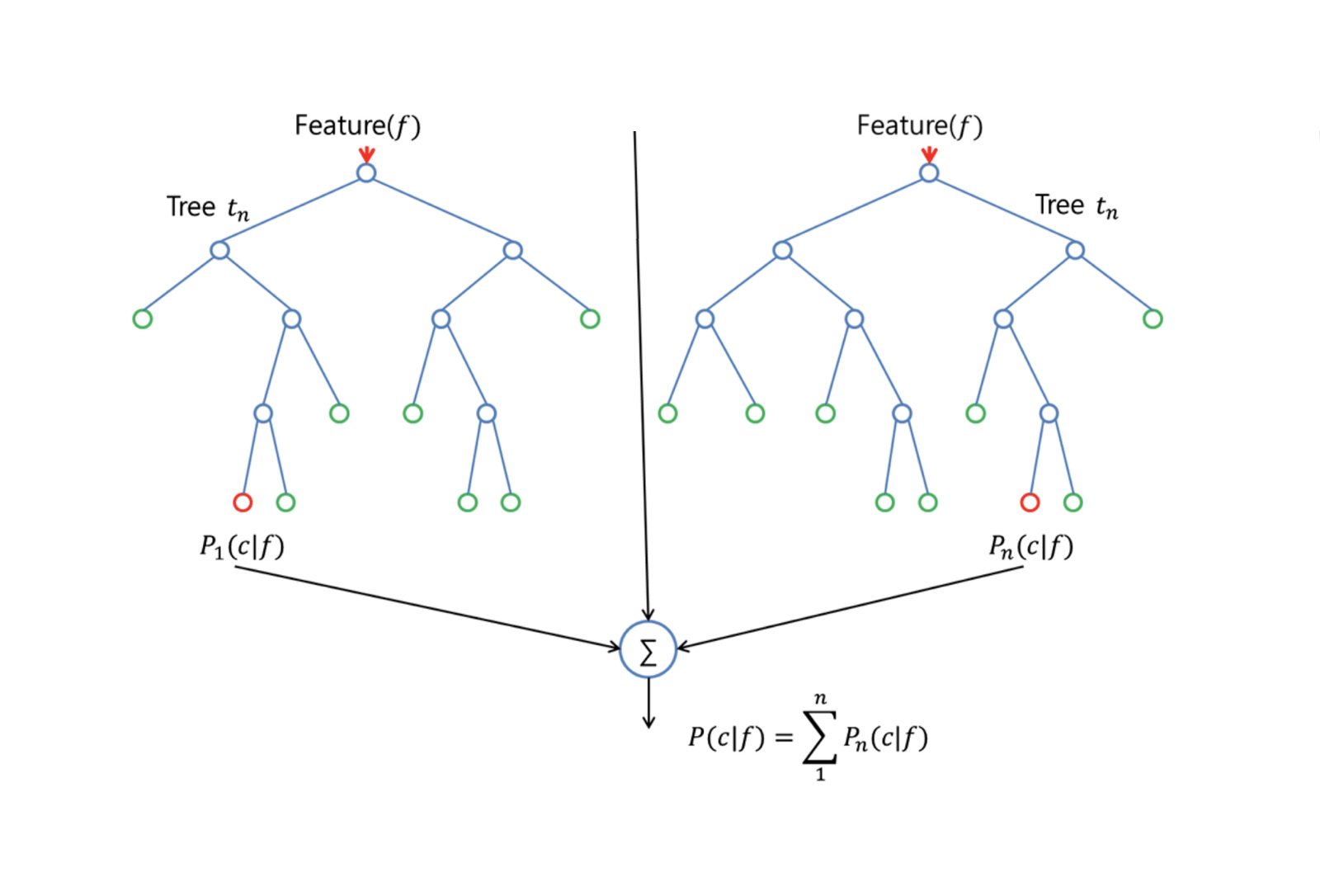In order to grow a random forest, you should:

• First assume that the number of cases in the training set is K. Then, take a random sample of these K cases, and then use this sample as the training set for growing the tree.

• If there are $p$ input variables, specify a number $m < p$ such that at each node, you can select $m$ random variables out of the $p$. The best split on these $m$ is used to split the node.

• Each tree is subsequently grown to the largest extent possible and no pruning is needed.

• Finally, aggregate the predictions of the target trees to predict new data.

Random Forests is very effective at estimating missing data and maintaining accuracy when a large proportions of the data is missing. It can also balance errors in datasets where the classes are imbalanced. Most importantly, it can handle massive datasets with large dimensionality. However, one disadvantage of using Random Forests is that you might easily overfit noisy datasets, especially in the case of doing regression.

### Boosting

Boosting is another approach to improve the predictions resulting from a decision tree. Like bagging and random forests, it is a general approach that can be applied to many statistical learning methods for regression or classification. Recall that bagging involves creating multiple copies of the original training dataset using the bootstrap, fitting a separate decision tree to each copy, and then combining all of the trees in order to create a single predictive model. Notably, each tree is built on a bootstrapped dataset, independent of the other trees.

Boosting works in a similar way, except that the trees are grown sequentially: each tree is grown using information from previously grown trees. Boosting does not involve bootstrap sampling; instead, each tree is fitted on a modified version of the original dataset.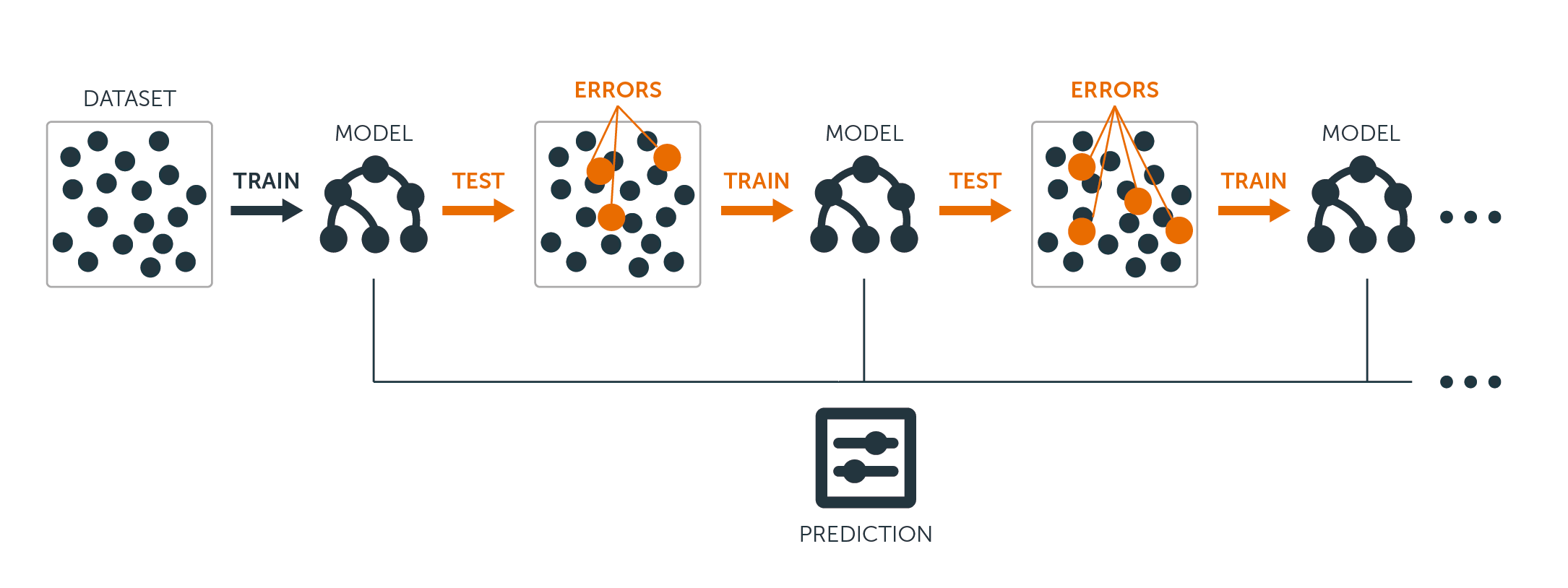For both regression and classification trees, boosting works like this:

• Unlike fitting a single large decision tree to the data, which amounts to fitting the data hard and potentially overfitting, the boosting approach instead learns slowly.

• Given the current model, you fit a decision tree to the residuals from the model. That is, you fit a tree using the current residuals, rather than the outcome $Y$, as the response.

• You then add this new decision tree into the fitted function in order to update the residuals. Each of these trees can be rather small, with just a few terminal nodes, determined by the parameter $d$ in the algorithm. By fitting small trees to the residuals, you slowly improve $\hat{f}$ in areas where it does not perform well.

• The shrinkage parameter $\nu$ slows the process down even further, allowing more and different shaped trees to attack the residuals.

Boosting is very useful when you have a lot of data and you expect the decision trees to be very complex. Boosting has been used to solve many challenging classification and regression problems, including risk analysis, sentiment analysis, predictive advertising, price modeling, sales estimation and patient diagnosis, among others.

## Decision Trees in R

### Classification Trees

For this part, you work with the Carseats dataset using the tree package in R. Mind that you need to install the ISLR and tree packages in your R Studio environment first. Let's first load the Carseats dataframe from the ISLR package.

library(ISLR)
data(package="ISLR")
carseats<-Carseats


Let's also load the tree package.

require(tree)


The Carseats dataset is a dataframe with 400 observations on the following 11 variables:

• Sales: unit sales in thousands

• CompPrice: price charged by competitor at each location

• Income: community income level in 1000s of dollars

• Advertising: local ad budget at each location in 1000s of dollars

• Population: regional pop in thousands

• Price: price for car seats at each site

• ShelveLoc: Bad, Good or Medium indicates quality of shelving location

• Age: age level of the population

• Education: ed level at location

• Urban: Yes/No

• US: Yes/No

names(carseats)


Let's take a look at the histogram of car sales:

hist(carseats$Sales)  Observe that Sales is a quantitative variable. You want to demonstrate it using trees with a binary response. To do so, you turn Sales into a binary variable, which will be called High. If the sales is less than 8, it will be not high. Otherwise, it will be high. Then you can put that new variable High back into the dataframe. High = ifelse(carseats$Sales<=8, "No", "Yes")
carseats = data.frame(carseats, High)


Now let's fill a model using decision trees. Of course, you can't have the Sales variable here because your response variable High was created from Sales. Thus, let's exclude it and fit the tree.

tree.carseats = tree(High~.-Sales, data=carseats)


Let's see the summary of your classification tree:

summary(tree.carseats)


You can see the variables involved, the number of terminal nodes, the residual mean deviance, as well as the misclassification error rate. To make it more visual, let's plot the tree as well, then annotate it using the handy text function:

plot(tree.carseats)
text(tree.carseats, pretty = 0)


There are so many variables, making it very complicated to look at the tree. At least, you can see that at each of the terminal nodes, they're labeled Yes or No. At each splitting node, the variables and the value of the splitting choice are shown (for example, Price < 92.5 or Advertising < 13.5).

For a detailed summary of the tree, simply print it. It'll be handy if you want to extact details from the tree for other purposes:

tree.carseats


It's time to prune the tree down. Let's create a training set and a test by splitting the carseats dataframe into 250 training and 150 test samples. First, you set a seed to make the results reproducible. Then you take a random sample of the ID (index) numbers of the samples. Specifically here, you sample from the set 1 to n row number of rows of car seats, which is 400. You want a sample of size 250 (by default, sample uses without replacement).

set.seed(101)
train=sample(1:nrow(carseats), 250)


So now you get this index of train, which indexes 250 of the 400 observations. You can refit the model with tree, using the same formula except telling the tree to use a subset equals train. Then let's make a plot:

tree.carseats = tree(High~.-Sales, carseats, subset=train)
plot(tree.carseats)
text(tree.carseats, pretty=0)


The plot looks a bit different because of the slightly different dataset. Nevertheless, the complexity of the tree looks roughly the same.

Now you're going to take this tree and predict it on the test set, using the predict method for trees. Here you'll want to actually predict the class labels.

tree.pred = predict(tree.carseats, carseats[-train,], type="class")


Then you can evalute the error by using a misclassification table.

with(carseats[-train,], table(tree.pred, High))


On the diagonals are the correct classifications, while off the diagonals are the incorrect ones. You only want to recored the correct ones. To do that, you can take the sum of the 2 diagonals divided by the total (150 test observations).

(72 + 43) / 150


Ok, you get an error of 0.76 with this tree.

When growing a big bushy tree, it could have too much variance. Thus, let's use cross-validation to prune the tree optimally. Using cv.tree, you'll use the misclassification error as the basis for doing the pruning.

cv.carseats = cv.tree(tree.carseats, FUN = prune.misclass)
cv.carseats


Printing out the results shows the details of the path of the cross-validation. You can see the sizes of the trees as they were pruned back, the deviances as the pruning proceeded, as well as the cost complexity parameter used in the process.

Let's plot this out:

plot(cv.carseats)


Looking at the plot, you see a downward spiral part because of the misclassification error on 250 cross-validated points. So let's pick a value in the downward steps (12). Then, let's prune the tree to a size of 12 to identify that tree. Finally, let's plot and annotate that tree to see the outcome.

prune.carseats = prune.misclass(tree.carseats, best = 12)
plot(prune.carseats)
text(prune.carseats, pretty=0)


It's a bit shallower than previous trees, and you can actually read the labels. Let's evaluate it on the test dataset again.

tree.pred = predict(prune.carseats, carseats[-train,], type="class")
with(carseats[-train,], table(tree.pred, High))

(74 + 39) / 150


Seems like the correct classifications dropped a little bit. It has done about the same as your original tree, so pruning did not hurt much with respect to misclassification errors, and gave a simpler tree.

Often case, trees don't give very good prediction errors, so let's go ahead take a look at random forests and boosting, which tend to outperform trees as far as prediction and misclassification are concerned.

### Random Forests

For this part, you will use the Boston housing data to explore random forests and boosting. The dataset is located in the MASS package. It gives housing values and other statistics in each of 506 suburbs of Boston based on a 1970 census.

library(MASS)
data(package="MASS")
boston<-Boston
dim(boston)
names(boston)


Let's also load the randomForest package.

require(randomForest)


To prepare data for random forest, let's set the seed and create a sample training set of 300 observations.

set.seed(101)
train = sample(1:nrow(boston), 300)


In this dataset, there are 506 surburbs of Boston. For each surburb, you have variables such as crime per capita, types of industry, average # of rooms per dwelling, average proportion of age of the houses etc. Let's use medv - the median value of owner-occupied homes for each of these surburbs, as the response variable.

Let's fit a random forest and see how well it performs. As being said, you use the response medv, the median housing value (in $1K dollars), and the training sample set. rf.boston = randomForest(medv~., data = boston, subset = train) rf.boston  Printing out the random forest gives its summary: the # of trees (500 were grown), the mean squared residuals (MSR), and the percentage of variance explained. The MSR and % variance explained are based on the out-of-bag estimates, a very clever device in random forests to get honest error estimates. The only tuning parameter in a random Forests is the argument called mtry, which is the number of variables that are selected at each split of each tree when you make a split. As seen here, mtry is 4 of the 13 exploratory variables (excluding medv) in the Boston Housing data - meaning that each time the tree comes to split a node, 4 variables would be selected at random, then the split would be confined to 1 of those 4 variables. That's how randomForests de-correlates the trees. You're going to fit a series of random forests. There are 13 variables, so let's have mtry range from 1 to 13: • In order to record the errors, you set up 2 variables oob.err and test.err. • In a loop of mtry from 1 to 13, you first fit the randomForest with that value of mtry on the train dataset, restricting the number of trees to be 350. • Then you extract the mean-squared-error on the object (the out-of-bag error). • Then you predict on the test dataset (boston[-train]) using fit (the fit of randomForest). • Lastly, you compute the test error: mean-squared error, which is equals to mean( (medv - pred) ^ 2 ). oob.err = double(13) test.err = double(13) for(mtry in 1:13){ fit = randomForest(medv~., data = boston, subset=train, mtry=mtry, ntree = 350) oob.err[mtry] = fit$mse
pred = predict(fit, boston[-train,])
test.err[mtry] = with(boston[-train,], mean( (medv-pred)^2 ))
}


Basically you just grew 4550 trees (13 times 350). Now let's make a plot using the matplot command. The test error and the out-of-bag error are binded together to make a 2-column matrix. There are a few other arguments in the matrix, including the plotting character values (pch = 23 means filled diamond), colors (red and blue), type equals both (plotting both points and connecting them with the lines), and name of y-axis (Mean Squared Error). You can also put a legend at the top right corner of the plot.

matplot(1:mtry, cbind(test.err, oob.err), pch = 23, col = c("red", "blue"), type = "b", ylab="Mean Squared Error")
legend("topright", legend = c("OOB", "Test"), pch = 23, col = c("red", "blue"))


Ideally, these 2 curves should line up, but it seems like the test error is a bit lower. However, there's a lot of variability in these test error estimates. Since the out-of-bag error estimate was computed on one dataset and the test error estimate was computed on another dataset, these differences are pretty much well within the standard errors.

Notice that the red curve is smoothly above the blue curve? These error estimates are very correlated, because the randomForest with mtry = 4 is very similar to the one with mtry = 5. That's why each of the curves is quite smooth. What you see is that mtry around 4 seems to be the most optimal choice, at least for the test error. This value of mtry for the out-of-bag error equals 9.

So with very few tiers, you have fitted a very powerful prediction model using random forests. How so? The left-hand side shows the performance of a single tree. The mean squared error on out-of-bag is 26, and you've dropped down to about 15 (just a bit above half). This means you reduced the error by half. Likewise for the test error, you reduced the error from 20 to 12.

### Boosting

Compared to random forests, boosting grows smaller and stubbier trees and goes at the bias. You will use the package GBM (Gradient Boosted Modeling), in R.

require(gbm)


GBM asks for the distribution, which is Gaussian, because you'll be doing squared error loss. You're going to ask GBM for 10,000 trees, which sounds like a lot, but these are going to be shallow trees. Interaction depth is the number of splits, so you want 4 splits in each tree. Shrinkage is 0.01, which is how much you're going to shrink the tree step back.

boost.boston = gbm(medv~., data = boston[train,], distribution = "gaussian", n.trees = 10000, shrinkage = 0.01, interaction.depth = 4)
summary(boost.boston)


The summary function gives a variable importance plot. It seems like there are 2 variables that have high relative importance: rm (number of rooms) and lstat (percentage of lower economic status people in the community). Let's plot these 2 variables:

plot(boost.boston,i="lstat")
plot(boost.boston,i="rm")


The 1st plot shows that the higher the proportion of lower status people in the suburb, the lower the value of the housing prices. The 2nd plot shows the reversed relationship with the number of rooms: the average number of rooms in the house increases as the price increases.

It's time to predict a boosted model on the test dataset. Let's look at the test performance as a function of the number of trees:

• First, you make a grid of number of trees in steps of 100 from 100 to 10,000.

• Then, you run the predict function on the boosted model. It takes n.trees as an argument, and produces a matrix of predictions on the test data.

• The dimensions of the matrix are 206 test observations and 100 different predict vectors at the 100 different values of tree.

n.trees = seq(from = 100, to = 10000, by = 100)
predmat = predict(boost.boston, newdata = boston[-train,], n.trees = n.trees)
dim(predmat)


It's time to compute the test error for each of the predict vectors:

• predmat is a matrix, medv is a vector, thus (predmat - medv) is a matrix of differences. You can use the apply function to the columns of these square differences (the mean). That would compute the column-wise mean squared error for the predict vectors.

• Then you make a plot using similar parameters to that one used for Random Forest. It would show a boosting error plot.

boost.err = with(boston[-train,], apply( (predmat - medv)^2, 2, mean) )
plot(n.trees, boost.err, pch = 23, ylab = "Mean Squared Error", xlab = "# Trees", main = "Boosting Test Error")
abline(h = min(test.err), col = "red")


The boosting error pretty much drops down as the number of trees increases. This is an evidence showing that boosting is reluctant to overfit. Let's also include the best test error from the randomForest into the plot. Boosting actually gets a reasonable amount below the test error for randomForest.

## Conclusion

So that's the end of this R tutorial on building decision tree models: classification trees, random forests, and boosted trees. The latter 2 are powerful methods that you can use anytime as needed. In my experience, boosting usually outperforms RandomForest, but RandomForest is easier to implement. In RandomForest, the only tuning parameter is the number of trees; while in boosting, more tuning parameters are required besides the number of trees, including the shrinkage and the interaction depth.

If you would like to learn more, be sure to take a look at our Machine Learning Toolbox course for R.